Chapter Notes: Electricity

# Electricity Chapter Notes - Science Class 10

 Table of contentsWhat is Electricity?Electric Current and CircuitElectric Potential and Potential DifferenceCircuit DiagramSymbols of Some Commonly Used Components in CircuitOhm’s LawFactors on which the Resistance of a Conductor dependsPractical Applications of Heating Effect of Electric Current## What is Electricity?

Electricity is a fundamental form of energy resulting from the movement of electric charges. It is characterized by the flow of electrons through conductors such as wires.

•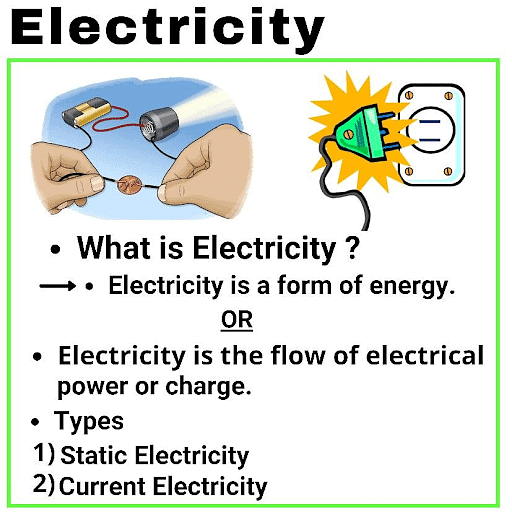Electricity powers various devices and systems, enabling them to perform functions like generating light, producing heat, and operating machinery.
• It is a crucial aspect of modern life and technology, with applications ranging from powering homes and appliances to driving electronic devices and industrial processes.

### What is an Electric Charge?

Charge is a fundamental particle in an atom. It may be positive or negative.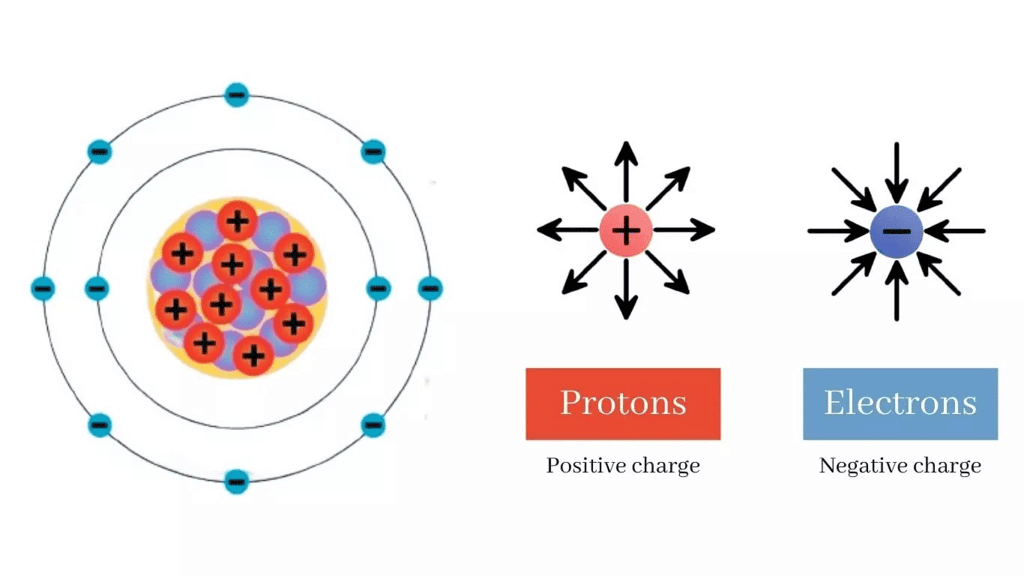Electric Charges: Positive and Negative

• Like charges repel each other.
• Unlike charges attract each other.
• Coulomb (C): S. I. unit of charge
• 1 Coulomb charge = Charge present on approx. 6 × 1018 electrons
• Charge on 1 electron = Negative charge of 1.6 × 10-19 C
i.e. Q = ne
Where, Q = Charge (total)
n = No. of electrons
e = Charge on 1 electron

## Electric Current and Circuit

Electric current is vital for powering technology, enabling safety and convenience, and facilitating energy distribution. Circuits are essential pathways that control the flow of current, playing a key role in electronics, medical applications, transportation, research, and sustainability efforts. They are fundamental to modern life, impacting almost every aspect of our daily existence.

### What is Electric Current?

Electric Current (I)  is the flow of electric charge through a conductor, such as a wire.

Current = Charge/Time or,

I = Q/T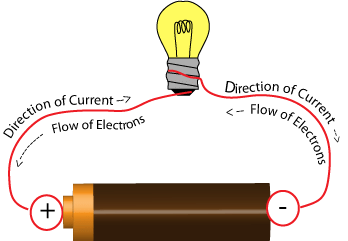Electric Current is rate of flow of Charge

S. I. unit of current = Ampere (A). An ampere is a unit of measure of the rate of electron flow or current in an electrical conductor. One ampere of current represents one coulomb of electrical charge (6.24 x 1018 charge carriers) moving past a specific point in one second.

⇒ 1 A = 1 Cs-1
⇒ 1 mA = 10-3 A
⇒ 1 µA = 10-6 A

• Current is measured by Ammeter. Its symbol is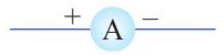• Ammeter has low resistance and always connected in series.
• Direction of current is taken opposite to flow of electrons as electrons were not known at the time when the phenomenon of electricity was discovered first and current was considered to be flow of positive charge.
Question for Chapter Notes: Electricity
Try yourself:A current of 0.5 A is drawn by a filament of an electric bulb for 10 minutes. What is the amount of electric charge that flows through the circuit?

## Electric Potential and Potential Difference

Electric potential and potential difference are fundamental concepts in the field of electricity and electromagnetism. They are used in various ways, both in theoretical understanding and practical applications.

What is Electric Potential?

• Electric potential is the work done in carrying a unit positive charge from infinity to a point.
• If W is the work done and q is the charge, then electric potential:

V=W/q

• The SI unit of electric potential is Volts (V).

What is Potential Difference ?

• Potential difference is Work done to move a unit charge from one point to another.
V = W/Q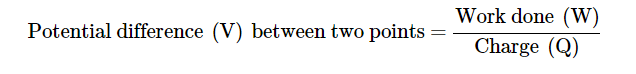What is meaning of 1 volt potential difference?

• S. I. unit of Potential difference is Volt (V)
1 V = 1 JC-1
• When 1 joule work is done in carrying one Coulomb charge then potential difference is called 1 volt.
• Voltmeter: It is an instrument to measure the potential difference. It has high resistance and always connected in parallel. Symbol is:• Current always flows from higher potential to lower potential.

### What is an Electric Circuit?

An electric circuit is a closed loop or pathway.It allows electric current to flow through various components.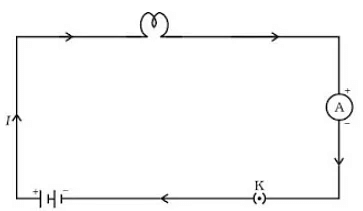Electric Circuit

• Components can include wires, switches, resistors, and energy sources.
• Electrons typically move through conductors, creating the flow of electric current.
• To maintain current flow, the circuit must be closed without interruptions.
• Used in various applications, from lighting to powering electronic devices.

## Circuit Diagram

A circuit diagram is a graphical representation of an electric circuit. In this diagram, various components of the circuit, such as cells (or batteries), plug keys, electrical components, and connecting wires, are symbolically represented using conventional symbols.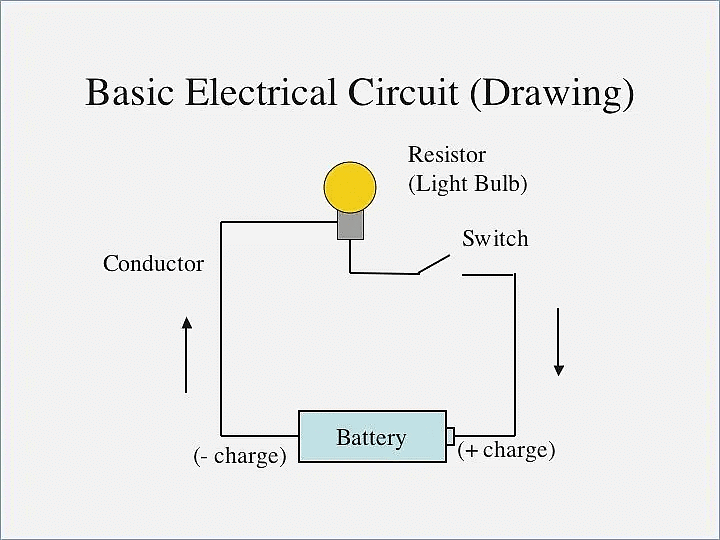•  These symbols are standardized and commonly used to simplify the visual representation of complex circuits, making it easier for engineers, technicians, and students to understand and work with electrical circuits.

• Circuit components are often represented by symbols in schematic diagrams.

##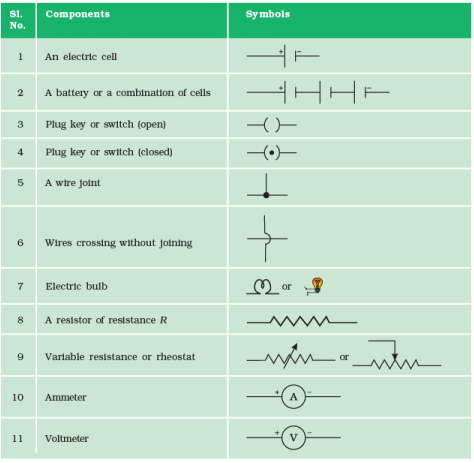### Ohm’s Law

Ohm's Law States that Potential difference across the two points of a metallic conductor is directly proportional to current passing through the circuit provided that temperature remains constant.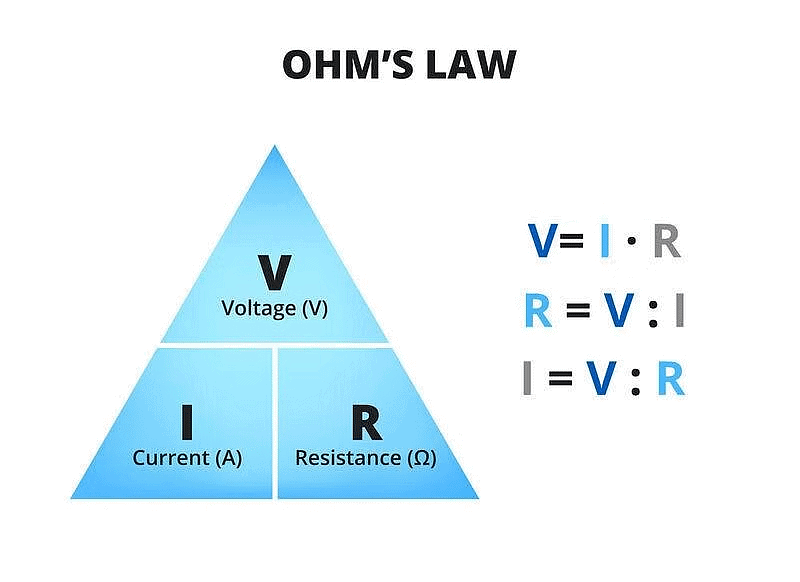• Mathematical expression for Ohm’s law
V ∝ I
⇒ V = IR
• R is a constant called resistance for a given metal.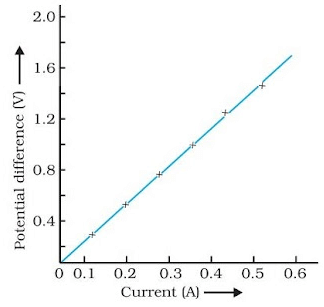V-I graph for Ohm’s law

• Resistance (R): It is the property of a conductor to resist the flow of charges through it.
• Ohm (Ω): S. I. unit of resistance.
• 1 ohm = 1 volt/1ampere.
When potential difference is 1 V and current through the circuit is 1 A, then resistance is 1 ohm.
• Rheostat: Variable resistance is a component used to regulate current without changing the source of voltage.

### Factors on which the Resistance of a Conductor depends

Resistance of a uniform metallic conductor is:
(i) directly proportional to the length of conductor,
(ii) inversely proportional to the area of cross-section,
(iii) directly proportional to the temperature and
(iv) depend on nature of material.

What is Resistivity (P)?
Resistivity is defined as the resistance offered by a cube of a material of side 1m when current flows perpendicular to its opposite faces.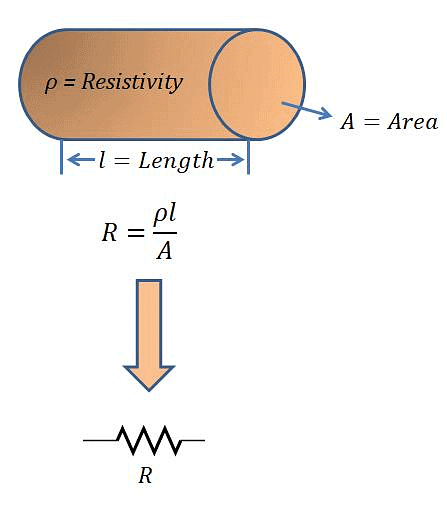• S.I. unit of  Resistivity is ohm-metre (Ωm).
• Resistivity does not change with change in length or area of cross-section but it changes with change in temperature.
• Range of resistivity of metals and alloys is 10-8 to 10-6 Ωm.
• Range of resistivity of insulators is 1012 to 1017 Ωm.
• Resistivity of alloy is generally higher than that of its constituent metals.
• Alloys do not oxidize (burn) readily at high temperature, so they are commonly used in electrical heating devices.
• Copper and aluminium are used for electrical transmission lines as they have low resistivity.

Question for Chapter Notes: Electricity
Try yourself:The potential difference between the terminals of an electric heater is 60 V when it draws a current of 4 A from the source. What current will the heater draw if the potential difference is increased to 120 V?

### Resistors in Series

When two or more resistors are connected end to end, the arrangement is called series combination.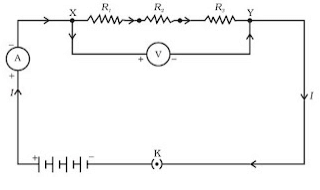Resistors in Series

Total/resultant/overall/effective resistance in series

Rs = R1 + R2 + R3

• Current through each resistor is same.
• Equivalent resistance is larger than the largest individual resistance.
• Total voltage = Sum of voltage drops
V = V1 + V2 + V3

Voltage across each resistor

• V1 = IR1
• V2 = IR2 [V1 + V2 + V3 = V]
• V3 = IR3V = IR

⇒ V = IR1 + IR2 + IR3
∝ IR = I(R1 + R2 + R3)
∝ R = R1 + R2 + R3

### Resistors in Parallel

When multiple resistors are connected in parallel in an electrical circuit, they are arranged so that both ends of each resistor are connected to the same two points, forming multiple paths for current flow.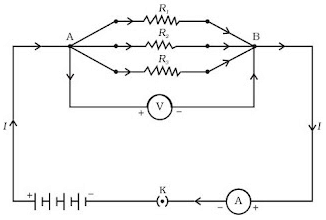Resisters in Parallel

• Voltage across each resistor is same and equal to the applied voltage.
• Total current is equal to sum of currents through the individual reistances.
I = I1 + I2 + I3
⇒ V/R = V/R1 + V/R2 + V/R3
• Reciprocal of equivalent resistance is equal to sum of reciprocals of individual resistances.
1/Rp = 1/R1 + 1/R2 + 1/R3
• Equivalent resistance is less than the value of the smallest individual resistance in the combination.

### Advantages of Parallel Combination over Series Combination

(i) In series circuit, when one component fails, the circuit is broken and none of the component works.
(ii) Different appliances have different requirement of current. This cannot be satisfied in series as current remains same.
(iii) The total resistance in a parallel circuit is decreased.

### Heating Effect of Electric Current:

If an electric circuit is purely resistive, the source of energy continually get dissipated entirely in form of heat. This is known as heating effect of electric current.
As E = P×T ∝  VIt  {E = H}
Heat produced, H = VIt  {V = IR}
Or, Heat produced, H = I2Rt

Question for Chapter Notes: Electricity
Try yourself:100 J of heat is produced each second in a 4 Ω resistance. What is the potential difference across the resistor?

## Practical Applications of Heating Effect of Electric Current

The heating effect of electric current, also known as Joule heating, is a fundamental concept in physics and electrical engineering. It's the phenomenon where electrical energy is converted into heat energy when current flows through a conductor with resistance. This heating effect has numerous practical applications in various fields.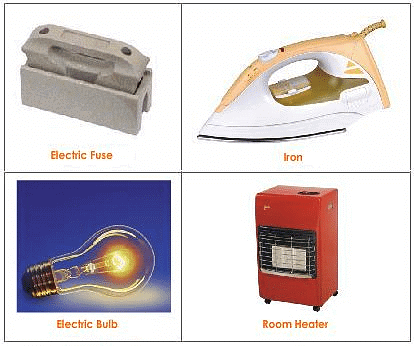Uses of Heating Effect of Electric Current

Joule’s Law of Heating Effect of Electric Current

It states that the heat produced in a resistor is (i) directly proportional to square of current, H ∝ I2

• It is directly proportional to resistance for a given current, H ∝ R
• It is directly proportional to time for which current flows through the conductor, H ∝ t.
So, H =  I2Rt
• Heating effect is desirable in devices like electric heater, electric iron, electric bulb, electric fuse, etc.
• Heating effect is undesirable in devices like computers, computer monitors (CRT), TV, refrigerators etc.
• In electric bulb, most of the power consumed by the filament appears a heat and a small part of it is radiated in form of light.

Filament of electric bulb is made up of tungsten because:
(i) it does not oxidise readily at high temperature.
(ii) it has high melting point (3380º C).

• The bulbs are filled with chemically inactive gases like nitrogen and argon to prolong the life of filament.

Electric Fuse: It is a safety device that protects our electrical appliances in case of short circuit or overloading.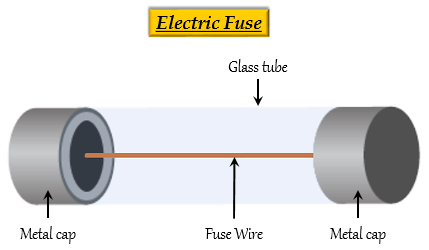• Fuse is made up of pure tin or alloy of copper and tin.
• Fuse is always connected in series with live wire.
• Fuse has low melting point.
• Current capacity of fuse is slightly higher than that of the appliance.

Electric Power: The rate at which electric energy is consumed or dissipated in an electric circuit.

P = VI
⇒ P = I2R = V2/R
S.I. unit of power = Watt (W)
⇒ 1 Watt = 1 volt × 1 ampere

Commercial unit of electric energy  = Kilo Watt hour (KWh)
⇒ 1 KWh = 3.6 × 106 J
⇒ 1 KWh = 1 unit of electric energy

The document Electricity Chapter Notes | Science Class 10 is a part of the Class 10 Course Science Class 10.
All you need of Class 10 at this link: Class 10

## Science Class 10

78 videos|509 docs|153 tests

## FAQs on Electricity Chapter Notes - Science Class 10

 1. What is electricity?Ans. Electricity is a form of energy resulting from the existence of charged particles (such as electrons or protons), either statically as an accumulation of charge or dynamically as a current.
 2. What is electric current and circuit?Ans. Electric current refers to the flow of electric charge in a conductor, while a circuit is a closed path or loop through which electric current can flow. A circuit typically consists of a power source (such as a battery), conductive wires, and various components (such as resistors or light bulbs) connected in a closed loop.
 3. What is electric potential and potential difference?Ans. Electric potential refers to the amount of electric potential energy per unit charge at a particular point in an electric field. Potential difference, on the other hand, is the difference in electric potential between two points in a circuit. It represents the work done per unit charge to move a charge from one point to another within the circuit.
 4. What are the symbols of some commonly used components in a circuit?Ans. Some commonly used symbols in circuit diagrams include: - Resistor: represented by a zigzag line - Battery: represented by a long line with a positive and negative terminal - Light bulb: represented by a circle with a cross inside - Switch: represented by a line with a gap that can be closed or opened - Ammeter: represented by the letter "A" inside a circle - Voltmeter: represented by the letter "V" inside a circle
 5. What are the factors on which the resistance of a conductor depends?Ans. The resistance of a conductor depends on the following factors: - Length: The longer the conductor, the higher the resistance. - Cross-sectional area: The larger the cross-sectional area, the lower the resistance. - Material: Different materials have different resistivities, which affect the resistance. - Temperature: The resistance of most conductors increases with temperature. - Presence of impurities or defects: Impurities or defects in the conductor can increase its resistance.

## Science Class 10

78 videos|509 docs|153 testsExplore Courses for Class 10 examSignup to see your scores go up within 7 days! Learn & Practice with 1000+ FREE Notes, Videos & Tests.
10M+ students study on EduRev
Track your progress, build streaks, highlight & save important lessons and more!
Related Searches

,

,

,

,

,

,

,

,

,

,

,

,

,

,

,

,

,

,

,

,

,

;